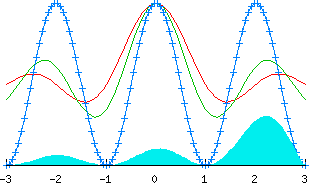# Gnuplot Tutorial1 GNUPLOT – version 4.0
Gnuplot is a free, command-driven,interactive, function and data plotting program. Pre-compiled, executeables and source code for Gnuplot 4.0 may be downloaded for Windows, OS2, DOS, and Linux.
The important enhancements provided by version 4.0 are described here.
On Unix/Linux systems start Gnuplot by simply typing:

` gnuplot`

For help on any topic type help followed by the name of the topic.
Full documentation is provided here.
<!–
web-enabled Gnuplot interface.
–>

2. FUNCTIONS
In general, any mathematical expression accepted by C, FORTRAN, Pascal, or
BASIC may be plotted. The precedence of operators is determined by the
specifications of the C programming language.
The supported functions include:

```      __________________________________________________________
Function            Returns
-----------      ------------------------------------------
abs(x)            absolute value of x, |x|
acos(x)           arc-cosine  of x
asin(x)           arc-sine    of x
atan(x)           arc-tangent of x
cos(x)            cosine      of x,  x is in radians.
cosh(x)           hyperbolic cosine of x, x is in radians
erf(x)            error function of x
exp(x)            exponential function of x, base e
inverf(x)         inverse error function of x
invnorm(x)        inverse normal distribution of x
log(x)            log of x, base e
log10(x)          log of x, base 10
norm(x)           normal Gaussian distribution function
rand(x)           pseudo-random number generator
sgn(x)            1 if x > 0, -1 if x < 0, 0 if x=0
sin(x)            sine      of x, x is in radians
sinh(x)           hyperbolic sine of x, x is in radians
sqrt(x)           the square root of x
tan(x)            tangent of x, x is in radians
tanh(x)           hyperbolic tangent of x, x is in radians
___________________________________________________________
Bessel, gamma, ibeta, igamma, and lgamma functions are also
supported.  Many functions can take complex arguments.
Binary and unary operators are also supported.

```

The supported operators in Gnuplot are the same as the corresponding
operators in the C programming language, except that most operators accept
integer, real, and complex arguments. The ** operator (exponentiation) is
supported as in FORTRAN. Parentheses may be used to change the order of
evaluation. The variable names x, y, and z are used as the default independent
variables.

3. THE plot AND splot COMMANDS
plot and splot are the primary commands in Gnuplot. They plot
functions and data in many many ways. plot is used to plot 2-d functions
and data, while splot plots 3-d surfaces and data.

```Syntax:
plot {[ranges]}
{[function] | {"[datafile]" {datafile-modifiers}}}
{axes [axes] } { [title-spec] } {with [style] }
{, {definitions,} [function] ...}

```

where either a [function] or the name of a data file enclosed in quotes is
supplied. For more complete descriptions, type: help plot help plot with help
plot using or help plot smooth .
3.1 Plotting Functions
To plot functions simply type: plot [function] at the gnuplot> prompt.
For example, try:

```      gnuplot>  plot sin(x)
gnuplot>  splot sin(x*y/20)
gnuplot>  plot sin(x) title 'Sine Function', tan(x) title 'Tangent'
```

3.2 Plotting Data
Discrete data contained in a file can be displayed by specifying the name of
the data file (enclosed in quotes) on the plot or splot command
line. Data files should have the data arranged in columns of numbers. Columns
should be separated by white space (tabs or spaces) only, (no commas). Lines
beginning with a # character are treated as comments and are ignored by Gnuplot.
A blank line in the data file results in a break in the line connecting data
points.
For example your data file, force.dat , might look like:

```      # This file is called   force.dat
# Force-Deflection data for a beam and a bar
# Deflection    Col-Force       Beam-Force
0.000              0              0
0.001            104             51
0.002            202            101
0.003            298            148
0.0031           290            149
0.004            289            201
0.0041           291            209
0.005            310            250
0.010            311            260
0.020            280            240
```

You can display your data by typing:

```      gnuplot>  plot  "force.dat" using 1:2 title 'Column',
"force.dat" using 1:3 title 'Beam'

```

Do not type blank space after the line continuation character, “” .
Your
data may be in multiple data files. In this case you may make your plot by using
a command like:

```      gnuplot>  plot  "fileA.dat" using 1:2 title 'data A',
"fileB.dat" using 1:3 title 'data B'
```

For information on plotting 3-D data, type:

```      gnuplot>  help splot datafile
```